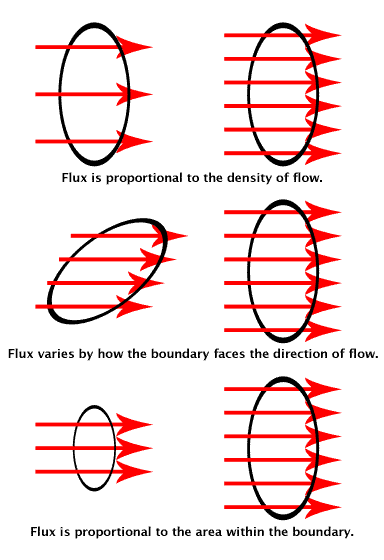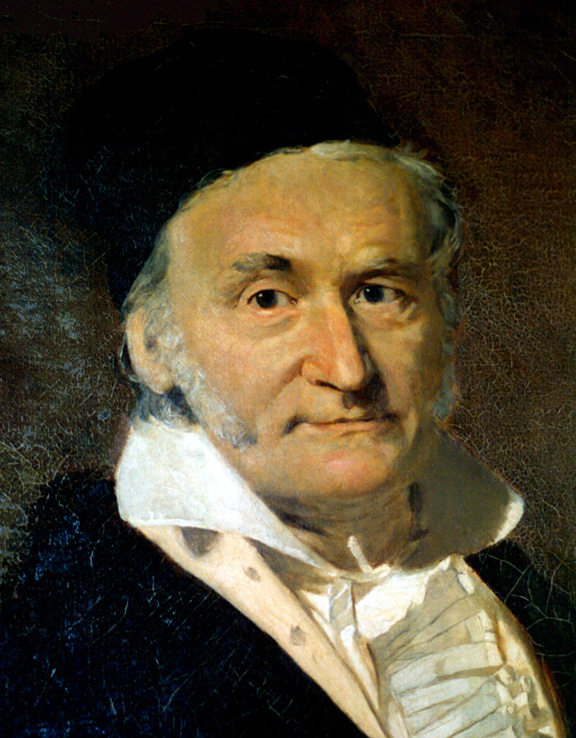## Electric Flux

Electric flux is the rate of flow of the electric field through a given area.

### Learning Objectives

Express the electric flux for uniform and non-uniform electric fields

### Key Takeaways

#### Key Points

• If the electric field is uniform, the electric flux passing through a surface of vector area S is $\Phi_\text{E} = \mathbf{\text{E}} \cdot \mathbf{\text{S}} = \text{ES} \cos \theta$.
• For a non-uniform electric field, the electric flux is.
• Electrical flux has SI units of volt metres (V m).

#### Key Terms

• electric field: A region of space around a charged particle, or between two voltages; it exerts a force on charged objects in its vicinity.

Electric flux is the rate of flow of the electric field through a given area (see ). Electric flux is proportional to the number of electric field lines going through a virtual surface.Electric Flux: Electric flux visualized. The ring shows the surface boundaries. The red arrows for the electric field lines.

If the electric field is uniform, the electric flux passing through a surface of vector area S is $\Phi_\text{E} = \mathbf{\text{E}} \cdot \mathbf{\text{S}} = \text{ES} \cos \theta$ where E is the magnitude of the electric field (having units of V/m), S is the area of the surface, and θ is the angle between the electric field lines and the normal ( perpendicular ) to S.

For a non-uniform electric field, the electric flux dΦE through a small surface area dS is given by $\text{d}\Phi_\text{E} = \mathbf{\text{E}} \cdot \text{d}\mathbf{\text{S}}$ (the electric field, E, multiplied by the component of area perpendicular to the field).

Gauss’ Law describes the electric flux over a surface S as the surface integral: $\Phi_\text{E} = \iint_\text{S} \mathbf{\text{E}} \cdot \text{d}\mathbf{\text{S}}$ where E is the electric field and dS is a differential area on the closed surface S with an outward facing surface normal defining its direction.

It is important to note that while the electric flux is not affected by charges that are not within the closed surface, the net electric field, E, in the Gauss’ Law equation, can be affected by charges that lie outside the closed surface. While Gauss’ Law holds for all situations, it is only useful for “by hand” calculations when high degrees of symmetry exist in the electric field. Examples include spherical and cylindrical symmetry.

Electric flux has SI units of volt metres (V m), or, equivalently, newton metres squared per coulomb (N m2 C−1). Thus, the SI base units of electric flux are kg·m3·s−3·A−1.

## Gauss’s Law

Gauss’s law is a law relating the distribution of electric charge to the resulting electric field.

### Learning Objectives

Describe relationship between the Gauss’s law and the Coulomb’s law

### Key Takeaways

#### Key Points

• Gauss’s law is one of the four Maxwell’s equations which form the basis of classical electrodynamics.
• Gauss’s law can be used to derive Coulomb ‘s law, and vice versa.
• Gauss’s law states that: The net outward normal electric flux through any closed surface is proportional to the total electric charge enclosed within that closed surface.

#### Key Terms

• electric displacement field: A vector field that appears in Maxwell’s equations.
• electric charge: A quantum number that determines the electromagnetic interactions of some subatomic particles; by convention, the electron has an electric charge of -1 and the proton +1, and quarks have fractional charge.
• electric field: A region of space around a charged particle, or between two voltages; it exerts a force on charged objects in its vicinity.

Gauss’s law, also known as Gauss’s flux theorem, is a law relating the distribution of electric charge to the resulting electric field.

The law was formulated by Carl Friedrich Gauss (see ) in 1835, but was not published until 1867. It is one of the four Maxwell’s equations which form the basis of classical electrodynamics, the other three being Gauss’s law for magnetism, Faraday’s law of induction, and Ampère’s law with Maxwell’s correction.Carl Friedrich Gauss: Carl Friedrich Gauss (1777–1855), painted by Christian Albrecht Jensen

Gauss’s law can be used to derive Coulomb’s law, and vice versa. Note that since Coulomb’s law only applies to stationary charges, there is no reason to expect Gauss’s law to hold for moving charges based on this derivation alone. In fact, Gauss’s law does hold for moving charges, and in this respect Gauss’s law is more general than Coulomb’s law.

In words, Gauss’s law states that: The net outward normal electric flux through any closed surface is proportional to the total electric charge enclosed within that closed surface.

The law can be expressed mathematically using vector calculus in integral form and differential form, both are equivalent since they are related by the divergence theorem, also called Gauss’s theorem. Each of these forms in turn can also be expressed two ways: In terms of a relation between the electric field E and the total electric charge, or in terms of the electric displacement field D and the free electric charge.

Gauss’s law has a close mathematical similarity with a number of laws in other areas of physics, such as Gauss’s law for magnetism and Gauss’s law for gravity. In fact, any “inverse-square law” can be formulated in a way similar to Gauss’s law: For example, Gauss’s law itself is essentially equivalent to the inverse-square Coulomb’s law, and Gauss’s law for gravity is essentially equivalent to the inverse-square Newton’s law of gravity.